# CBSE Class 10 Maths Chapter 1 Real Numbers Objective Questions

Want to assess how thorough you are with the concepts of “Real Numbers”? Are you worried about how you would fare if asked MCQs from this chapter? With the CBSE Class 10 exam pattern undergoing a major overhaul for the coming academic year, you can now expect more objective type questions for the exams. So, we have compiled here some questions from the chapter and arranged them topic wise for your easy convenience. Find the CBSE Class 10 Maths Chapter 1 Real Numbers Objective Questions given in this article.

Our focus is on providing solutions that can simplify the complex fundamentals of the subject. We believe in giving access to quality solutions that can help you score high marks in the exams. Below is the link to download the CBSE Class 10 Maths Chapter 1 Real Numbers Objective Questions.

### List of Sub-Topics Covered In Real Numbers

The chapter discusses real numbers and their applications. It is an important topic of CBSE Class 10 Maths. While the topic is taught in Class 9 as well, in Class 10 it goes more in depth into the concept. Here, we have given the list of sub-topics that are covered in chapter 1 of CBSE Class 10 Maths Objective Questions. They are as follows:

1.1 Introduction to Real Numbers (We have 5 MCQs From This Topic)

1.2 Revisiting Irrational Numbers (We have 5 MCQs Covering This Topic)

1.3 Revisiting Rational Numbers and Their Decimal Expansions (5 MCQs Listed)

1.4 The Fundamental Theorem of Arithmetic (5MCQs Given From This Topic)

## Download Free CBSE Class 10 Maths Chapter 1 Real Numbers Objective Questions PDF

### Introduction to Real Numbers

1. What is the least number that must be added to 1056 so the number is divisible by 23?
1. 0
2. 3
3. 2
4. 1

Solution: We have,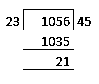On dividing 1056 by 23, we got 21 as remainder.

⇒ If we add 23 – 21 = 2 to the dividend 1056, we will get a number completely divisible by 23.

∴ Required number = (23 – 21) = 2

1. The difference of two numbers is 1365. On dividing the larger number by the smaller, we get 6 as quotient and the 15 as remainder. What is the smaller number?
1. 360
2. 295
3. 270
4. 240

Solution: Let the smaller number be x.

⇒ Larger number = (x+1365)

⇒x+1365=6x+15

⇒5x=1350

⇒x=270

∴ Larger number is (270 + 1365) = 1635

and smaller number is 270.

1. Euclid’s division lemma states “Given positive integers a and b, there exist unique integers q and r satisfying a=bq+r”. Which of the following is true for r?
1. r>a
2. r<0
3. 0≤r<b
4. r>b

Solution: Euclid’s division lemma:

Given positive integers a and b, there exist unique integers q and r satisfying a = bq + r where 0≤r<b.

Basically, it can be observed that remainder can never be more than the divisor, and is a non-negative integer (could be zero).

1. a and b, when divided by 7 and 6 respectively, leave remainders p and q respectively. What is the maximum value of p + q?
1. 5
2. 6
3. 12
4. 11

Solution: There exist integers m and n such that a = 7m + p and b = 6n + q such that

0<p<7⇒

Maximum value of p will be 6.

0<q<6⇒

Maximum value of p will be 5.

Therefore, the maximum value of p + q will be 11.

1. If HCF (1008, 20) = HCF (20, a) = HCF (a, b) where 1008=20×q+a; 20=a×m+b where (q, a) and (m, b) are positive integers satisfying Euclid’s Division Lemma. What could be the values of a and b?
1. 24, 8
2. 20, 8
3. 10, 4
4. 8, 4

Solution: If p=d×q+r, (p>q) where p, q, d, r are integers and for a given (p, d), there exist a unique (q, r), then HCF (p, d) = HCF (d, r). Because this relation holds true, the Euclid’s Division Algorithm exists in a step by step manner. So, to find the HCF (1008, 20), we use Euclid’s division lemma at every step.

Step 1: 1008=20×50+8 ⇒ HCF(1008, 20) = HCF(20,8) ⇒ a could be 8

Step 2: 20=8×2+4 ⇒ HCF(20, 8) = HCF(8,4) ⇒ b could be 4

Step 3: 8=4×2+0

HCF = 4

Since 1008=20×q+a where q and a are positive integers satisfy Euclid’s Division Lemma, we must have 0≤a<20. So a is surely 8 and b is 4.

### Revisiting Irrational Numbers

1. Which one of the following can’t be the square of a natural number?
1. 42437
2. 20164
3. 81225
4. 32761

Solutions: We know that the square of a natural number never ends in 2, 3, 7 and 8.

∴ 42437 can’t be the square of a natural number.

1. If the number 91876y2 is completely divisible by 8, then the smallest whole number in place of y will be:
1. 2
2. 4
3. 3
4. 1

Solution: For a number to be divisible by 8, the last three digits must be divisible by 8.

Here, 6y2 must be divisible by 8.

For y=3, 632 is divisible by 8.

1. If x and y are the two digits of the number 653xy such that this number is divisible by 80, then x + y =?
1. 4 or 8
2. 6
3. 4
4. 8

Solution: If the number is divisible by another number, it will be divisible by its factors too.

The factors of 80=2×5×8

For the number to be divisible by both 2 and 5, the last digit should be 0

So, y=0

So we can rewrite the number as 653×0

Now For the number to be divisible by 8; last 3 digits should be divisible by 8

So 3×0 should be divisible by 8

So, x can be either 2 or 6 since 320 and 360 are divisible by 8

If x=2; then the number becomes 65320 which is not divisible by 80

If x=6; then the number becomes 65360 which is divisible by 80

Hence the value of x=6

So, x+y=6+0 = 6

1. Which among the following options is irrational?
1. 3.1415926535… (non-repeating and non-terminating)
2. 10.2
3. (0.2)2
4. 0.2

Answer: (A) 3.1415926535… (Non-repeating and non-terminating)

Solution: Non-terminating and non-repeating decimals are irrational.

If the denominator of a rational number is in the form of  where n and m are non-negative integers, then the rational number is terminating.

0.2 = 2/ 10 = 2/ (2 x 5)

∴ 0.2 has terminating decimal and is a rational number.

3.1415926535… is non-terminating and non-repeating.

∴ 3.1415926535… is irrational.

1/0.2 = 10/2

∴ 1/ 0.2 has terminating decimal and is a rational number.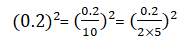(0.2)2 has terminating decimal and is a rational number.

1. ‘s’ is called irrational if it cannot be written in the form of _____ where p and q are integers and ______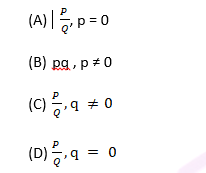Answer: (C) p/q, q ≠ 0

Solution: ‘s’ is called irrational if it cannot be written in the form of pq, where p and q are integers and q ≠ 0

### Revisiting Rational Numbers and Their Decimal Expansions

1. Write down the decimal expansions of :  13/ 6250
1. 0.0208
2. 0.00208
3. 0.00512
4. 0.00416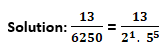To get the denominator in powers of 10, multiply both numerator and denominator by 24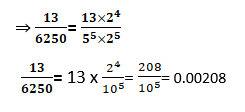1. Without actually performing the long division, state whether the following rational numbers will have a terminating decimal expansion or a non-terminating decimal expansion: 23/8
1. non-terminating non – repeating decimal
2. non-terminating repeating decimal
3. non-terminating decimal
4. terminating decimal

Solution: The given number is 23/8

We know a rational number is expressed in simplest form p/q and if q can be expressed as

×, then it is a terminating decimal

Clearly, 2 and 5 are not the factors of 23

8 can be expressed in terms of its primes as 2×2×2

Or, 8 =., where m = 3 and n = 0

Since q can be expressed in the form of., we can say 23/8 is a terminating decimal.

1. Without actually performing the long division, state whether the following rational number will have a terminating decimal expansion or a non-terminating decimal expansion: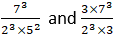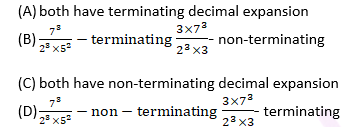Answer: (A)both have terminating decimal expansion

Solution: If the denominator of a rational number is in the form of., where m and n are non-negative integers, then the rational number has terminating decimal expansion.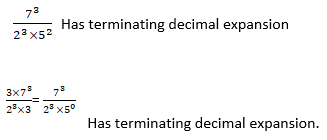1. Decide whether 52.123456789 is a rational number or not. If rational (in the form p/q), what can you say about the prime factors of q?
1. Rational Number, Prime factor of q will be only 2.
2. Rational Number, Prime factor of q will have a factor other than 2 or 5.
3. Not rational number
4. Rational Number, Prime factors of q will have either 2 or 5 or both

Answer: (D)Rational Number, Prime factors of q will have either 2 or 5 or both

Solution: It is rational because decimal expansion is terminating. Therefore, it can be expressed in p/q form where factors of q are of the form. and n and m are non-negative integers.

1. Let x= p/q  be a rational number, such that the prime factorization of q is not of the form, where n, m are non-negative integers. Then, x has a decimal expansion which is ____________
1. Terminating
2. Non-terminating non-repeating
3. Terminating non-repeating
4. Non-terminating repeating (recurring)

Solution: Non-terminating repeating (recurring)

### The Fundamental Theorem of Arithmetic

1. Find the HCF of 1848, 3058 and 1331.
1. 9
2. 14
3. 13
4. 11

Solution: Consider first two numbers 1848 and 3058, where 3058 > 1848.

3058 = 1848 × 1 + 1210

1848 = 1210 × 1 + 638

1210 = 638 × 1 + 572

638 = 572 × 1 + 66

572 = 66 × 8 + 44

66 = 44 × 1 + 22

44 = 22 × 2 + 0

∴ HCF of 1848 and 3058 is 22.

Let us find the HCF of the numbers 1331 and 22.

1331 = 22 × 60 + 11

22 = 11 × 2 + 0

HCF of 1331 and 22 is 11

∴ HCF of the three given numbers 1848, 3058 and 1331 is 11.

1. An army contingent of 616 members is to march behind an army band of 32 members in a parade. The two groups are to march in the same number of columns. What is the maximum number of columns in which they can march?
1. 8
2. 7
3. 6
4. 9

Solution: Maximum number of columns = HCF of 616 and 32

616= 23×7×11

32= 25

∴ HCF of 616 and 32 =  =8

1. Find the biggest number which can divide both 324 and 144.
1. 18
2. 36
3. 9
4. 21

Solution: Finding the biggest number that will divide both 324 and 144 is same as finding the HCF of both.

Prime factorizing the two numbers we get,

324=3×3×3×3×2×2

144=3×3×2×2×2×2

Now, taking the common factors between them gives us the HCF.

Therefore, HCF =2×2×3×3=36

1. Which of the following does not satisfy the following property?
A number which divides 542 and 128 and leaves a remainder 2 in both cases.
2. 12
3. 9
4. 6
5. 3

Solution: Suppose the number be x. Since it divides 542 and 128 leave a remainder 2, using the Euclid’s division algorithm for 542 and x, 542 = ax + 2, where a is an integer. So, ax = 542 – 2 = 540, i.e. x is a factor of 540. Similarly x is a factor of (128 – 2).

The HCF of 542 – 2 = 540, 128 – 2 = 126:

Step 1: 540=126×4+36

Step 2: 126=36×3+18

Step 3: 36=18×2+0

HCF is 18

3, 6, 9 are factors of 18. 12 is the only number which isn’t a factor and hence doesn’t satisfy the above property.

1. p =  (where q is a prime < 7) is the prime factorization representation of ‘p’. What is the value of p?
1. p = 700
2. p = 900
3. p = 36
4. can’t say